Catch up on the latest AI articlesThe Bi-directional Convolution Pooling "LiftPool" Is Here!

3 main points
✔️ Proposal of bi-directional pooling method "LiftPool"
✔️ Avoids missing information in pooling process based on classical signal processing theory
✔️
Excellent performance and robustness in various tasks such as image classification and segmentation

written by Jiaojiao ZhaoCees G. M. Snoek
(Submitted on 29 Sept 2020 (modified: 24 Feb 2021))

Subjects: bidirectional, poolingcode：First of all

Pooling in CNNs is a very important operation because it widens the receptive field and improves the robustness to input variations. However, the downsampling of feature maps by pooling is a process that loses a lot of information, and the lost information cannot be recovered.

In the paper presented in this article, we propose LiftPool, a bidirectional pooling method that addresses such missing information and unidirectionality.

LiftPool is capable of both down pooling (LiftDownPool) and up pooling (LiftUpPool). Furthermore, it achieves excellent performance in image classification and segmentation and is highly robust to input corruption and perturbations.

Technique

LiftPool is composed of two parts: down pooling (LiftDownPool) and up pooling (LiftUpPool). The LiftPool is inspired by the Lifting Scheme in signal processing, where the LiftDownPool decomposes the input into four sub-bands (LL, LH, HL, HH), and the LiftUpPool is designed to perform appropriate up-sampling based on these sub-bands. The LiftUpPool is designed to perform appropriate upsampling based on these subbands.

The overall image is as follows.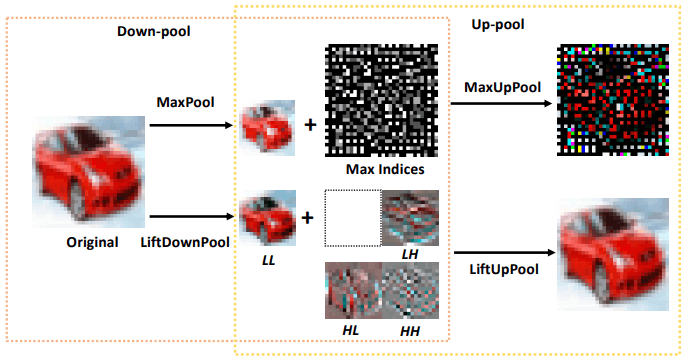Note that when MaxPool→MaxUpPool is applied, the original image cannot be restored properly, whereas when LiftDownPool→LiftUpPool is applied, the original image can be restored properly. Next, we will explain about LiftDownPool and LiftUpPool respectively.

LiftDownPool(one-dimensional case)

As an example, we consider a one-dimensional signal. LiftDownPool decomposes the given signal $x=[x_1,x_2,x_3,... ,x_n], x_n \in R$ is decomposed into a downscaled approximate signal $s$ and a difference signal $d$.

$s,d=F(x)$

Here, $F(⋅)$ consists of three functions $f_{split},f_{predict}, and f_{update}$. In the one-dimensional case, LiftDownPool is shown in the following figure (a).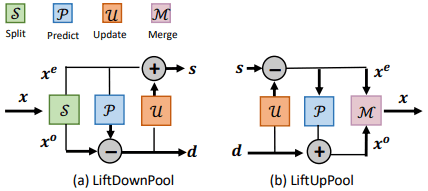The details of the split, predict, and update is as follows.

Split

The split function is represented by $f_{split}: x \mapsto (x^e,x^o)$. In this case, the given signal $x$ has even index $x^e=[x_2,x_4,... ,x_{2k}]$ and odd index $x^o=[x_1,x_2,... ,x_{2k+1}]$, which is divided into two sets.

・Predict

The predict function is represented by $f_{predict}: (x^e,x^o) \mapsto d$. In this case, the predictor $P(⋅)$, given one set (e.g. $x^e$), predicts the other set ($x^o$). In this case, the difference signal $D$ is defined as follows.

$d=x^o-P(x^e)$

In this case, $d$ is the high-frequency component.

・Update

The update function is represented by $f_{update}: (x^e,d) \mapsto s$. It adds $U(d)$ to the downsampled $x^e$ from the input signal $x$ and generates an approximate signal $s$ by smoothing.

$s=x^e+U(d)$

In this case, $S$ is the low-frequency component. Also, $P(⋅)$ and $U(⋅)$ are designed from ReLU and convolution operations as follows.

$P(-) = Conv(kernel=1,stride=1,groups=C) ◦ ReLU() ◦ Conv(kernel=K,stride=1,groups=C)$

$U(-) = Conv(kernel=1,stride=1,groups=C) ◦ ReLU() ◦ Conv(kernel=K,stride=1,groups=C)$

$K$ denotes the kernel size and $C$ denotes the number of input channels. In LiftPool, these functions are trained end-to-end by a deep neural network.

Two constraint terms $c_u,c_p$ are then added to the final loss function, and the loss is represented by the following equation

$L=L_{task}+\lambda_uc_u+\lambda_pc_p$

$L_task$ represents the loss of a specific task, such as a classification task or a segmentation task.

The $C_U$ is defined to minimize the L2-norm between $S$ and $X^o$ by the following equation.

$c_u=||s-x^o|||_2=||U(d)+x^e-x^o||_2$

$C_P$ is defined by the following equation to minimize $D$.

$c_p=||x^o-P(x^e)||_2$

LiftDownPool(2D)

In LiftDownPool in 2D, we first run LiftDownPool-1D in the horizontal direction to obtain the horizontal low-frequency approximation $s$ and the horizontal high-frequency difference $d$.

Then, for each of these two, perform a vertical LiftDownPool-1D.

In this case, $s$ is decomposed into LL (vertical and horizontal low-frequency components) and LH (vertical low-frequency and horizontal high-frequency components), $d$ is decomposed into HL (vertical high-frequency and horizontal low-frequency components) and HH (vertical and horizontal high-frequency components), and four subbands are obtained.

An example of an actual feature map obtained is shown below.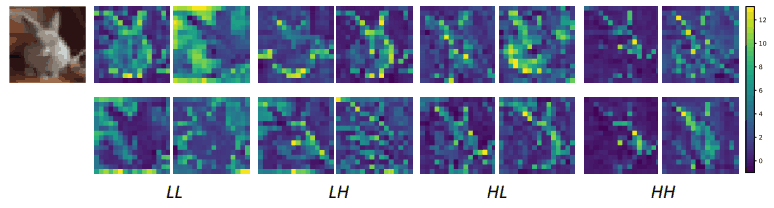In this case, LL denotes a smoothed feature map with low detail, and LH, HL, and HH denote feature maps with high detail along the horizontal, vertical, and diagonal directions, respectively.

The LiftDownPool can be generalized to any $n$-dimensional signal.

LiftUpPool

As an example again, consider a one-dimensional signal.

At this time, LiftUpPool generates an upsampled signal $x$ from $s,d$.

$x=G(s,d)$

Here, G consists of three functions $f_{update,f_{predict},f_{merge}}$. These processes can be expressed as $s,d \mapsto x^e,d \mapsto x^e,x^o \mapsto x$, and more specifically as follows (the overall image is shown in Figure (b) above).

$x^e=s-U(d) (update)$

$x^o=d+P(x^e) (predict)$

$x=f_{merge}(x^e,x^o) (merge)$

Up-pooling has been used in tasks such as segmentation, super-resolution, and image colorization. However, most of the existing pooling functions are not invertible, resulting in information loss when producing high-resolution output.

On the other hand, LiftUpPool can generate more detailed output by using multiple subbands generated by LiftDownPool.

Experiment

In our experiments, we apply LiftDownPool in the image classification task and LiftDownPool and LiftUpPool in the semantic segmentation task to verify the results.

・Flexibility in sub-band selection

LiftDownPool in 2D images can generate four sub-bands, each containing a different type of information, so you have the flexibility to choose which sub-bands to keep as a result of pooling.

To begin with, the Top-1 error when using VGG13 on CIFAR-100 is as follows.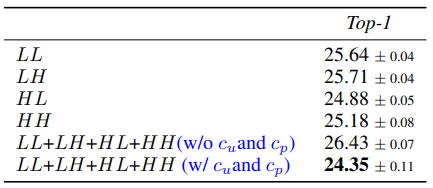In the case of image classification, the HL (vertical) subband has shown good performance.

We also find that summing and combining all subbands improves performance with little additional computational cost. Furthermore, we found that the constraints on $c_u$ and $c_p$ allow us to reduce the error.

・Kernel size and effect of LiftDownPool

In the following table, we show the performance of $P(⋅), U(⋅)$ against the kernel size.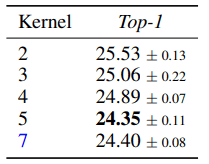The performance tends to improve gradually as the kernel size increases but does not improve when the kernel size reaches 7. Based on this result, we set the kernel size to 5 in subsequent experiments, and use all subbands in total. Next, a comparison between LiftDownPool and other pooling (MaxPool, AveragePool, Skip, etc.) is shown below.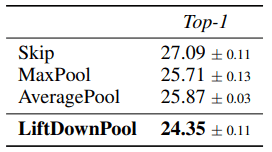As you can see, LiftDownPool outperformed the other pooling methods.

・Generalizability

Here are the comparison results when applying LiftDownPool (and various pooling methods) on the ImageNet dataset to backbones such as ResNet18/50 and MobileNet-V2.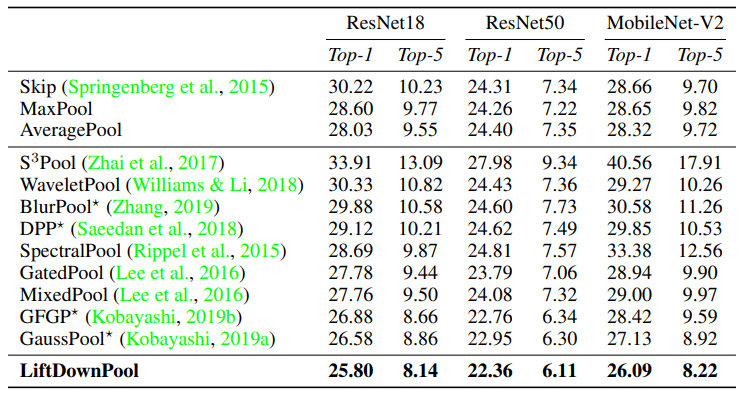It was shown that LiftDownPool outperforms the other methods on all backbone architectures.

In LiftPool, $P, U$ has a one-dimensional convolution process, which adds some trainable parameters. To investigate the efficiency of these parameters, we compare LiftPool with other parameterized pooling methods (GFGP, GaussPool) using ResNet50 on ImageNet, and the results are as follows.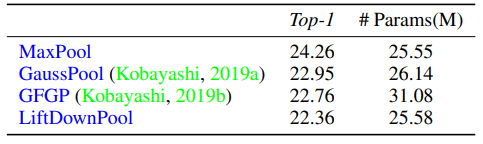Compared with MaxPool, the error rate is reduced by 1.9% while the increase in the number of parameters is kept to about 3K, which shows the effectiveness of the LiftPool scheme.

Robustness analysis

・non-distributional robustness

A good down pooling method is expected to be robust against perturbations and noise. Here, we use ResNet50 to investigate the robustness of LiftDownPool to corruptions on ImageNet-C or perturbations on ImageNet-P.

The results at this time are as follows.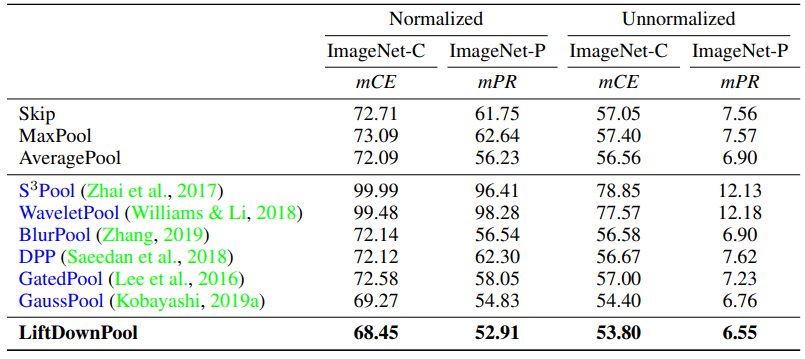LiftDownPool effectively reduces the mCE (mean Corruption Error) and mFR (mean Flip Rate) and shows high robustness against corruption and perturbations such as noise and blur.

・Distribution shift robustness

Following prior work, we evaluate how often the network outputs the same classification for the same image with two different distributions. The results for the various backbones trained on ImageNet are as follows.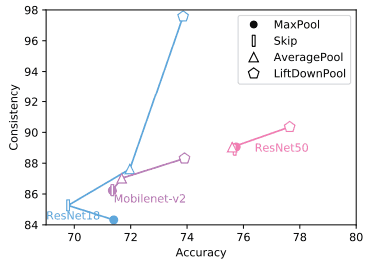Compared to other pooling methods, LiftDownPool (denoted by a pentagon) was found to improve both accuracy and consistency.

LiftPool in the Semantic Segmentation Task

For semantic segmentation on PASCAL-VOC12, we replace the pooling layer in SegNet with LiftDown/UpPool. In this case, LiftDownPool keeps only LL sub-bands, and LH/HL/HH sub-bands are used for up-sampled feature map generation. For comparison, the results of the same experiment for MaxUpPool and BlurPool are as follows.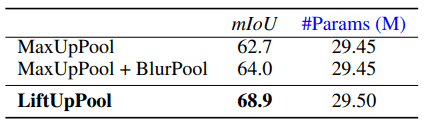While the increase in the number of parameters is slight, we see a significant improvement in performance from the baseline.

The actual generated example is shown below.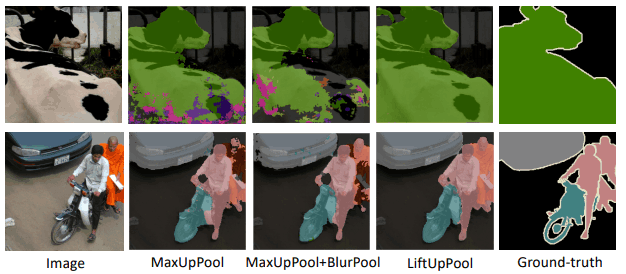In addition, when we apply LiftDownPool to DeepLabV3Plus-ResNet50 and run the semantic segmentation task, the results are as follows.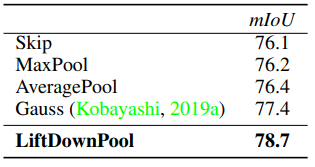Even in this case, all were shown to be significantly above baseline, indicating the effectiveness of LiftPool in all experiments.

Summary

The LiftPool presented in this article is a bidirectional pooling that can perform both down-pooling and up-pooling. Among the proposed LiftPools, the down-pooling LiftDownPool is shown to improve the image classification performance and robustness.

We also show that LiftUpPool, which is an up pooling method, significantly outperforms MaxUpPool in segmentation. Considering the fact that pooling is widely used in CNNs, the importance of LiftPool, which can significantly improve the performance by only changing the pooling process, is very high.

If you have any suggestions for improvement of the content of the article,